#### Exact methods

If the total number of stages is small, it is possible in practice to compute exact representations. Some methods are based on an observation that the cost-to-come is piecewise linear and convex . A linear-programming problem results, which can be solved using the techniques that were described for finding randomized saddle points of zero-sum games in Section 9.3. Due to the numerous constraints, methods have been proposed that dramatically reduce the number that need to be considered in some circumstances (see the suggested reading on POMDPs at the end of the chapter).

An exact, discrete representation can be computed as follows. Suppose that the initial condition space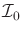consists of one initial condition,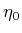(or a finite number of initial conditions), and that there are no more than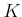stages at which decisions are made. Since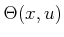and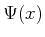are assumed to be finite, there is a finite number of possible final I-states,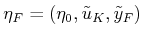. For each of these, the distribution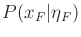can be computed, which is alternatively represented as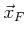. Following this, (12.4) is used to compute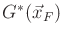for each possible. The number of these states is unfortunately exponential in the total number of stages, but at least there are finitely many. The dynamic programming recurrence (12.10) can be applied for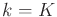to roll back one stage. It is known that each possible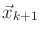will be a point in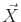at which a value was computed because values were computed for possible all I-states. Therefore, interpolation is not necessary. Equation 12.10 can be applied repeatedly until the first stage is reached. In each iteration, no interpolation is needed because the cost-to-go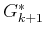was computed for each possible next I-state. Given the enormous size of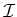, this method is practical only for very small problems.

Steven M LaValle 2020-08-14